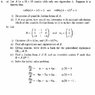# Jordan Forms and Eigenproblems

• shaon0

See attachment.

## The Attempt at a Solution

In a) ii); The use of a chain diagram is required but I have no idea how to produce one. As for i); I have no idea how to do this.

In b); (B+5I)v=(1,2,-1) and (B+5I)^2v=0. The eigenvalues are 5 (with multiplicity 2) and 2 (w/ AM of 1). Eigenvectors are (-1,-2,-1) and (-2,-2,1). For iii); I row reduced (B+5I) to get basis of {(0,1,2);(3,-1,1)} but I'm not sure that this is the way to go. I'm completely lost with iv) as I can't get P or J. But i'd think P contains the eigenvectors on the columns and J can be deduced from here by solving. I think v) I can do.

#### Attachments

•Eigenproblem.jpg
34.2 KB · Views: 421

Hi shaon0!

Not really sure what a chain diagram is.
Sounds like a nifty way to construct the generalized eigenvectors.

As for (a), how much do you know about constructing Jordan normal forms, and about constructing generalized eigenvectors?

For (b) you need to consider that (B+5I)^2v=0, which makes v a generalized eigenvector, and it makes v1=(B+5I)v a regular eigenvector.

How did you find the eigenvalue 2?
Actually, it should be -2.
Did you deduce it based on the trace of matrix B?

The matrix J is the Jordan normal form related to your 2 eigenvalues, for which you get 2 blocks.
Do you know how to define the blocks?
The matrix P is the matrix of the 3 generalized eigenvectors.

Hi shaon0!

Not really sure what a chain diagram is.
Sounds like a nifty way to construct the generalized eigenvectors.

As for (a), how much do you know about constructing Jordan normal forms, and about constructing generalized eigenvectors?

For (b) you need to consider that (B+5I)^2v=0, which makes v a generalized eigenvector, and it makes v1=(B+5I)v a regular eigenvector.

How did you find the eigenvalue 2?
Actually, it should be -2.
Did you deduce it based on the trace of matrix B?

The matrix J is the Jordan normal form related to your 2 eigenvalues, for which you get 2 blocks.
Do you know how to define the blocks?
The matrix P is the matrix of the 3 generalized eigenvectors.

Yes, sorry -2 is the other eigenvalue. It was a typo and yes i used the trace. J2(5)oJ1(-2) is the jordan form. Which I realize now. I'm not sure how to find the generalised eigenvectors. Are the three eigenvectors I have found, the generalised ones? What makes an eigenvector generalised?

Yes, sorry -2 is the other eigenvalue. It was a typo and yes i used the trace. J2(5)oJ1(-2) is the jordan form. Which I realize now. I'm not sure how to find the generalised eigenvectors. Are the three eigenvectors I have found, the generalised ones? What makes an eigenvector generalised?

Good!
And yes, the three vectors you found are the generalized eigenvectors.
Note that one of them is not an eigenvector.

The generalized eigenvectors are also the vectors that are not eigenvectors themselves, but that are a sort of 2nd or higher order eigenvectors.
That is, you find them from (B-λI)r=0, where r is the order of 2 or higher.

The matrix P is the matrix with the generalized eigenvectors as columns.

Take a look at this wiki page:
http://en.wikipedia.org/wiki/Jordan_normal_form
It explains in more detail what generalized eigenvectors are and how you find them.

Good!
And yes, the three vectors you found are the generalized eigenvectors.
Note that one of them is not an eigenvector.

The generalized eigenvectors are also the vectors that are not eigenvectors themselves, but that are a sort of 2nd or higher order eigenvectors.
That is, you find them from (B-λI)r=0, where r is the order of 2 or higher.

The matrix P is the matrix with the generalized eigenvectors as columns.

Take a look at this wiki page:
http://en.wikipedia.org/wiki/Jordan_normal_form
It explains in more detail what generalized eigenvectors are and how you find them.

Oh ok, right I've got it. Your explanations are very clear. Hopefully now I'll pass my linear algebra exam. Thanks again.

Oh ok, right I've got it. Your explanations are very clear. Hopefully now I'll pass my linear algebra exam. Thanks again.

So can you solve (a) now?

(Since understanding about generalized eigenvectors is a prerequisite for it.)